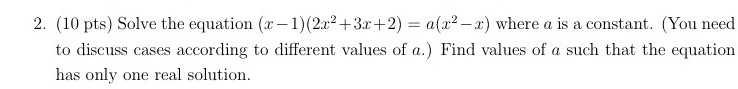### ¿Todavía tienes preguntas de matemáticas?

Pregunte a nuestros tutores expertos
Algebra
Pregunta2. (10 pts) Solve the equation $$( x - 1 ) ( 2 x ^ { 2 } + 3 x + 2 ) = a ( x ^ { 2 } - x )$$ where $$a$$ is a constant. (You need to discuss cases according to different values of $$a$$ .) Find values of $$a$$ such that the equation has only one real solution.